# 自然语言处理 10：分布语义学

## 墨尔本大学 COMP90042 课程笔记

Posted by YEY on April 2, 2020

# Lecture 10 分布语义学

## 1. 分布语义学

### 1.1 词汇数据库的问题

• 需要手工构建
• 成本高
• 人类的注解可能存在偏见和噪音
• 语言是动态的
• 新的单词：俚语、专业术语等等
• 新的词义（senses）
• 互联网为我们提供了大量的文本，我们可以利用它们获得单词含义吗？

### 1.2 分布假设

• You shall know a word by the company it keeps（你可以通过其周围的上下文单词来了解一个目标单词）” —— (Firth, 1957)
• 共现文档通常指示了主题（文档（document） 作为上下文）。
• 例如：$\textit{voting}$（投票）和 $\textit{politics}$（政治）
如果我们观察文档，会发现这两个单词经常出现在同一文档中。因此，不同单词的共现文档在一定程度上反映了这些单词在某种主题方面的关联。
• 局部上下文反映了一个单词的语义类别（单词窗口（word window） 作为上下文）。
• 例如：$\textit{eat a pizza, eat a burger}$
可以看到，$“\textit{pizza}”$ 和 $“\textit{burger}”$ 这两个单词都具有共同的局部上下文 $“\textit{eat a}”$，由此我们可以知道这两个单词都具有和 $“\textit{eat a}”$ 相关的某种含义。

### 1.3 根据上下文猜测单词含义

• 根据其用法来学习一个未知单词。

• 例如：现在我们有一个单词 $\textit{tezgüino}$，我们并不知道其含义，我们试图通过从该单词的一些用法中学习到其含义。
下面是该单词出现过的一些例句：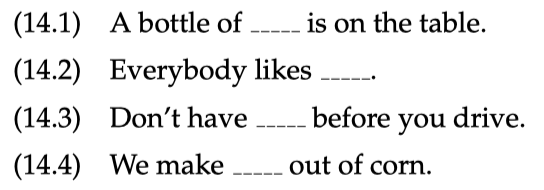作为人类，通过结合常识，我们可以大概猜测到 $\textit{tezgüino}$ 可能是某种含酒精饮品。

• 我们再查看一下在相同（或者类似）上下文中的其他单词的情况。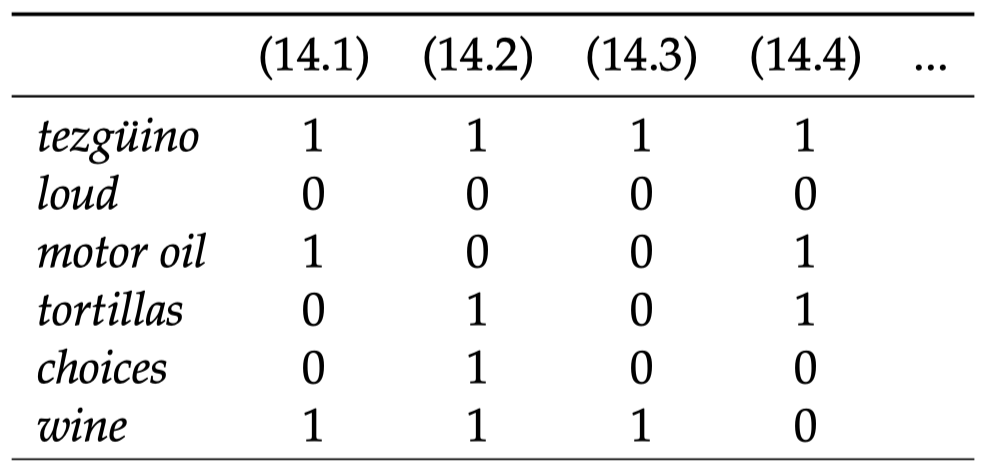可以看到，单词 $\textit{wine}$ 出现过的类似场景最多。因此，尽管我们并不知道 $\textit{tezgüino}$ 的具体含义，我们还是可以认为 $\textit{tezgüino}$ 和 $\textit{wine}$ 在单词含义方面非常相近。

### 1.4 词向量• 每一行都可以视为一个 词向量（word vector）
• 它描述了单词的分布特性（目标单词附近的上下文单词信息）。
• 捕获各种语义关系，例如：同义（synonymy）、类比（analogy）等。

### 1.5 词嵌入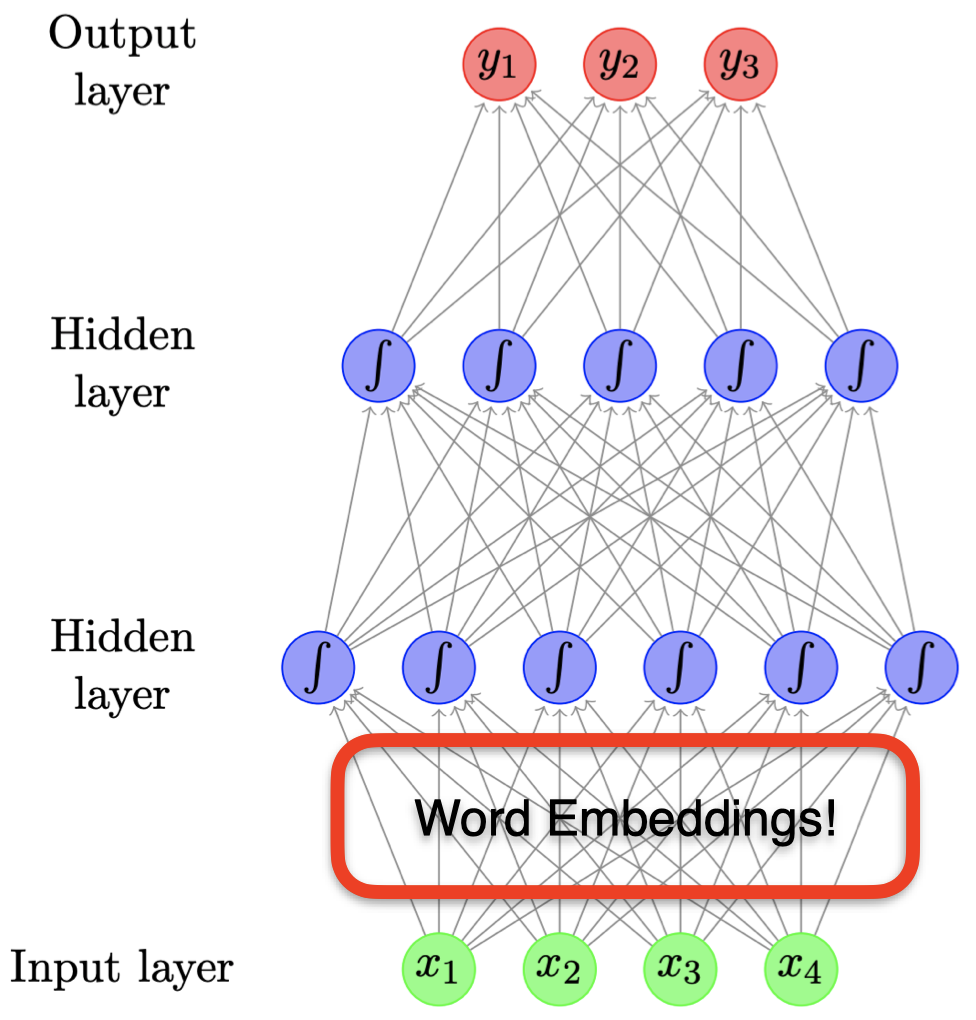• 在之前的神经网络的章节中，我们已经见过另一种词向量：词嵌入（word embeddings）
• 例如：在使用前馈神经网络进行文本分类时，第一层相当于是词嵌入层，该层的权重矩阵即词嵌入矩阵。
• 这里，我们将学习通过其他方法产生词向量：
• 基于计数的方法
• 专为学习词向量而设计的更高效的神经网络方法

## 2. 基于计数的方法

### 2.1 向量空间模型

• 基本思想：将单词含义表示为一个向量。
• 通常，我们将 文档（documents）视为上下文。
• 一个矩阵，两种视角：
• 一个文档由其所包含的单词表示
• 一个单词由其出现过的文档表示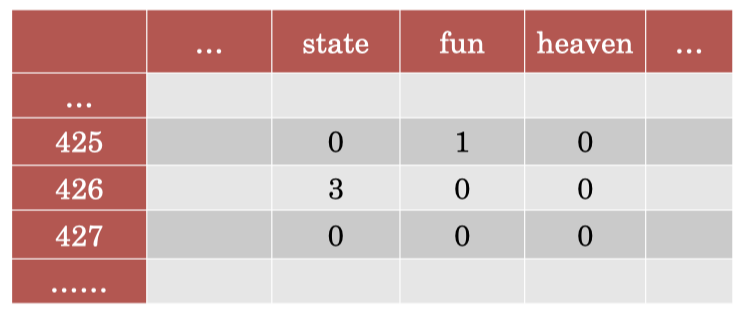这里，每一行都表示语料库中的一个文档，每一列表示语料库的词汇表中的一个单词，单元格中的数字表示该单词在对应文档中出现的频率。例如：单词 $\textit{state}$ 没有在文档 $425$ 中出现过，所以对应的值为 $0$，但是它在文档 $426$ 中出现过 $3$ 次，所以对应值为 $3$。

当我们构建完成这样一个矩阵后，我们可以从两种视角来看待它：如果我们观察每一行，我们可以将其视为每个文档的词袋模型表示；如果我们观察每一列，我们可以将其视为每个单词的词向量表示。

### 2.2 操作 VSM

• 给值进行加权（不仅是频率）
我们可以对矩阵中的值进行一些除了词频之外的更好的加权方式。
• 创建低维的密集向量
假如我们有非常多的文档，例如 100 万个，那么我们的词向量的维度也将会是 100 万维，因为每个维度都代表一个文档。但是，这样的话词向量的维度过高了，并且非常稀疏，其中大部分维度的值都是 $0$。
• 一旦我们完成了这些，我们就可以比较不同的词向量，并计算彼此之间的相似度等等。

### 2.3 TF-IDF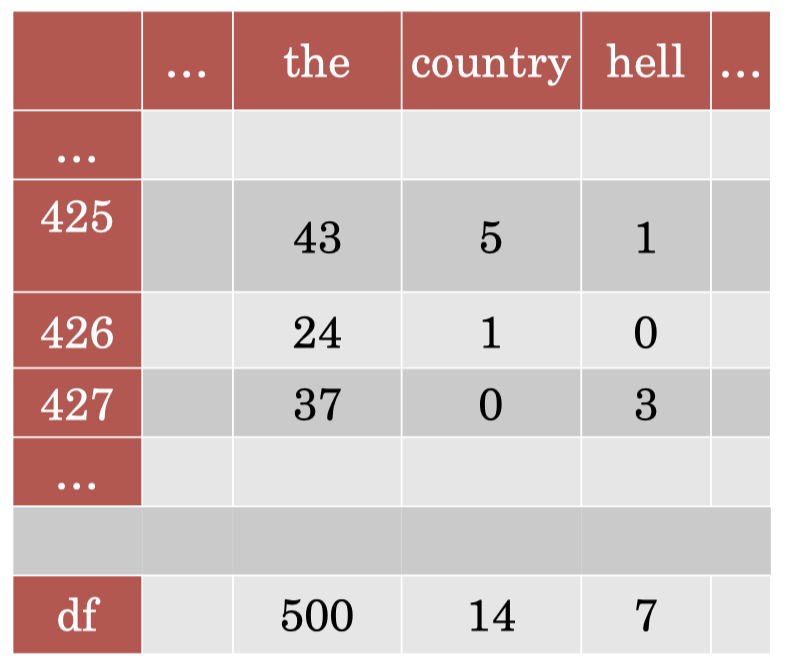TF 矩阵

$\textit{idf}_w=\log \dfrac{|D|}{\textit{df}_w}$

$\textit{idf}_\textit{the}=\log_2 \dfrac{500}{500}=\log_2 1=0$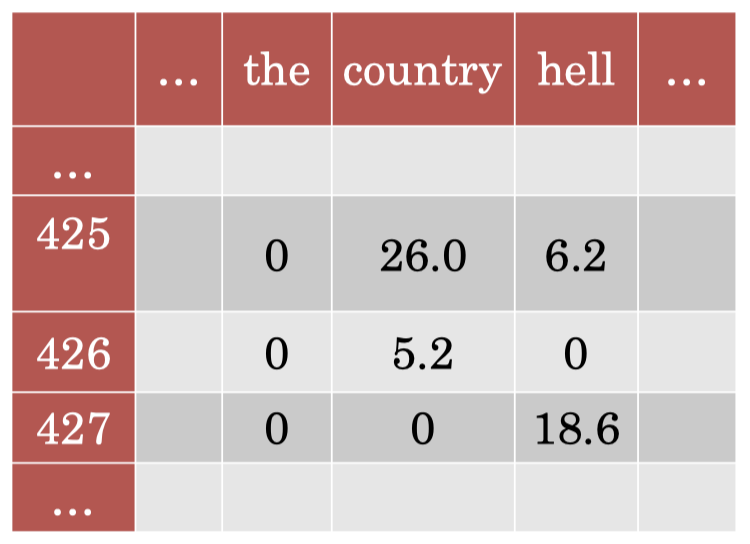TF-IDF 矩阵

TF-IDF 的核心思想在于：对于在大部分文档中都频繁出现的单词（例如：“$\textit{the}$”），我们给予更低的权重，因为它们包含的信息量很少。

### 2.4 降维

• Term-document 矩阵过于 稀疏（sparse）
• 降维（Dimensionality reduction）：创建更短、更密集的向量。
• 更实用（特征更少）
• 消除噪声（更好地避免过拟合）

### 2.5 奇异值分解（SVD）

SVD 的核心思想很简单：给定一个矩阵 $A$，我们可以将其分解为 3 个相乘的矩阵：$U$、$\Sigma$ 和 $V^{\mathrm T}$。

$A=U\Sigma V^{\mathrm T}$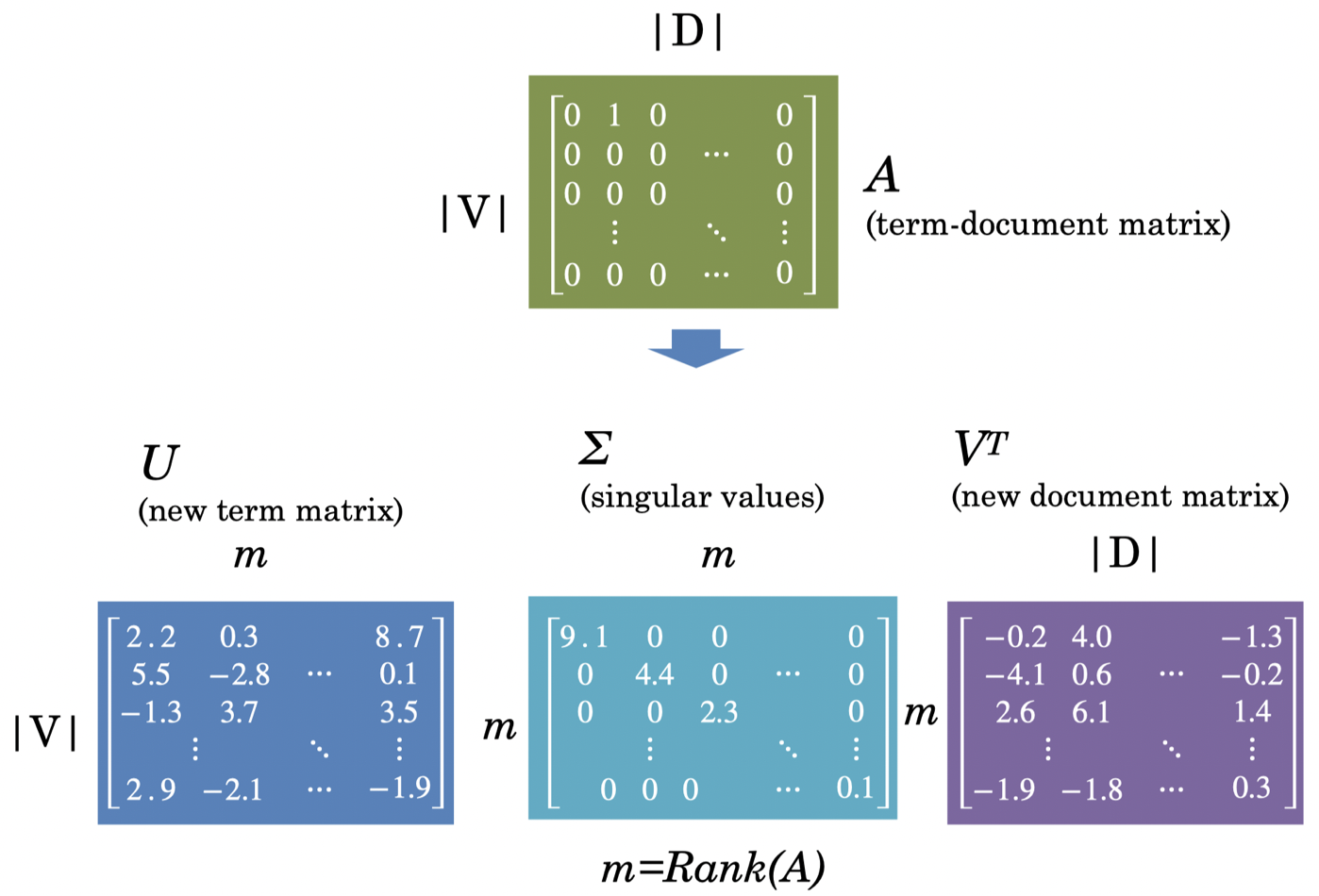• 原始矩阵 $A$ 是 term-document 矩阵（出现为 $1$，没有出现为$0$），其行数为词汇表大小 $|V|$，列数为文档总数 $|D|$。
• $U$ 是新的 term 矩阵，行数为词汇表大小 $|V|$，列数为 $m$。其中，$m$ 为矩阵 $A$ 的秩，即 $m=Rank(A)$。
• $\Sigma$ 是大小为 $m\times m$ 的奇异值矩阵，它是一个对角矩阵。
• $V^{\mathrm T}$ 是新的 document 矩阵，行数为 $m$，列数为文档总数 $|D|$。

### 2.6 截断：潜在语义分析（LSA）

• 将 $U$、$\Sigma$ 和 $V^{\mathrm T}$ 截断为 $k$ 个维度，从而生成原始矩阵的最佳 $k$ 阶近似。
• 因此，截断后的 $U_k$（或者 $V_k^{\mathrm T}$）是对应单词的一个新的低维表示。
• 通常，$k$ 的取值为 100-5000。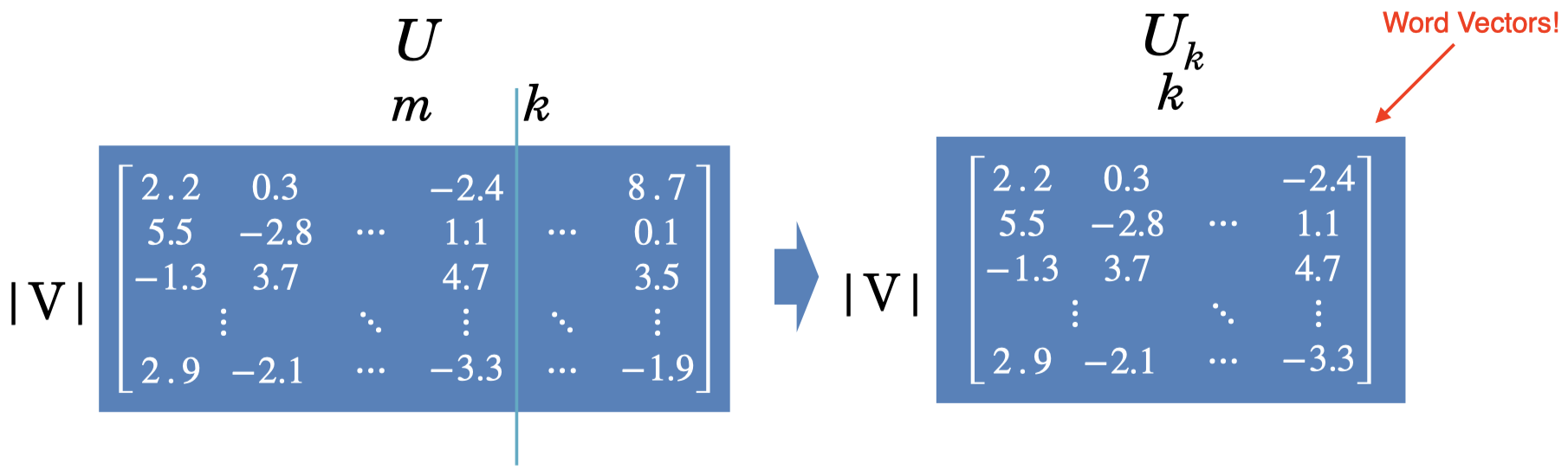### 2.7 单词作为上下文

• 构建一个矩阵，关于目标单词随着其他单词一起出现的频率。
• 在某些预定义的上下文中（通常是一个窗口）。
相比将文档作为上下文，我们可以选择目标单词附近固定范围内的某些单词作为上下文。

例如：我们可以选择窗口大小为 $5$，即目标单词前后长度为 $5$ 的范围内的单词作为上下文单词。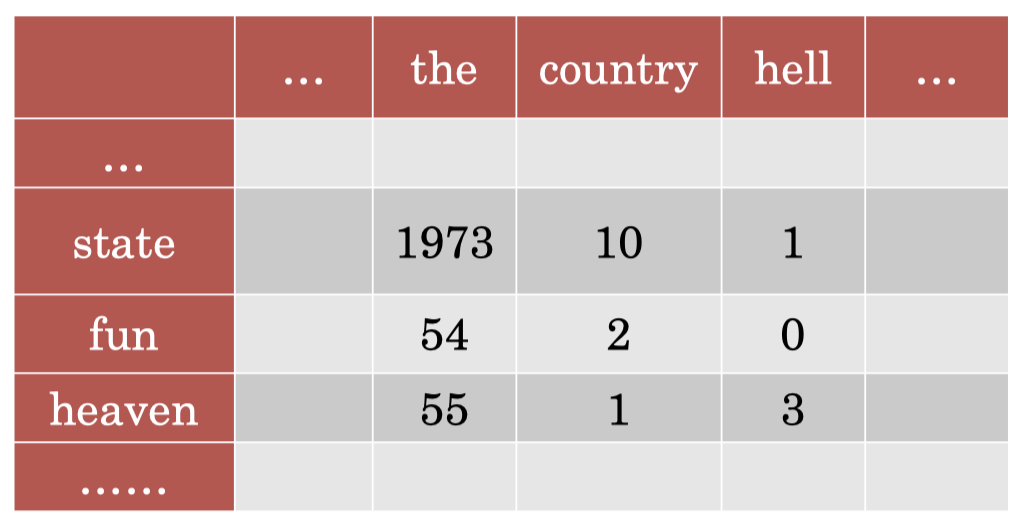可以看到，在上面的矩阵中，每一行都表示一个单词，每一列也表示一个单词。单元格中的数字表示目标单词和上下文单词在整个语料库中所有大小为 $5$ 的窗口内（即从语料库中提取所有的 five-grams）共同出现的频率。

• 但是，原始频率存在一个明显的问题：整个矩阵被常见单词所主导。
• 例如：我们可以看到，由于单词 “$\textit{the}$” 出现频率很高，使得对应单元格内的值非常大。之前，我们采用 TF-IDF 加权来给予常见单词的值一个折扣。但是这里，我们无法采用 TF-IDF，因为这里我们没有涉及到文档。相应地，这里我们可以采用点互信息（PMI）的方法来处理这个问题。

### 2.8 点互信息（PMI）

• 它们的联合概率分布
• 它们各自的概率分布（假设彼此之间互相独立）
$\textit{PMI}(x,y)=\log_2 \dfrac{p(x,y)}{p(x)p(y)}$

### 2.9 例子：计算 PMI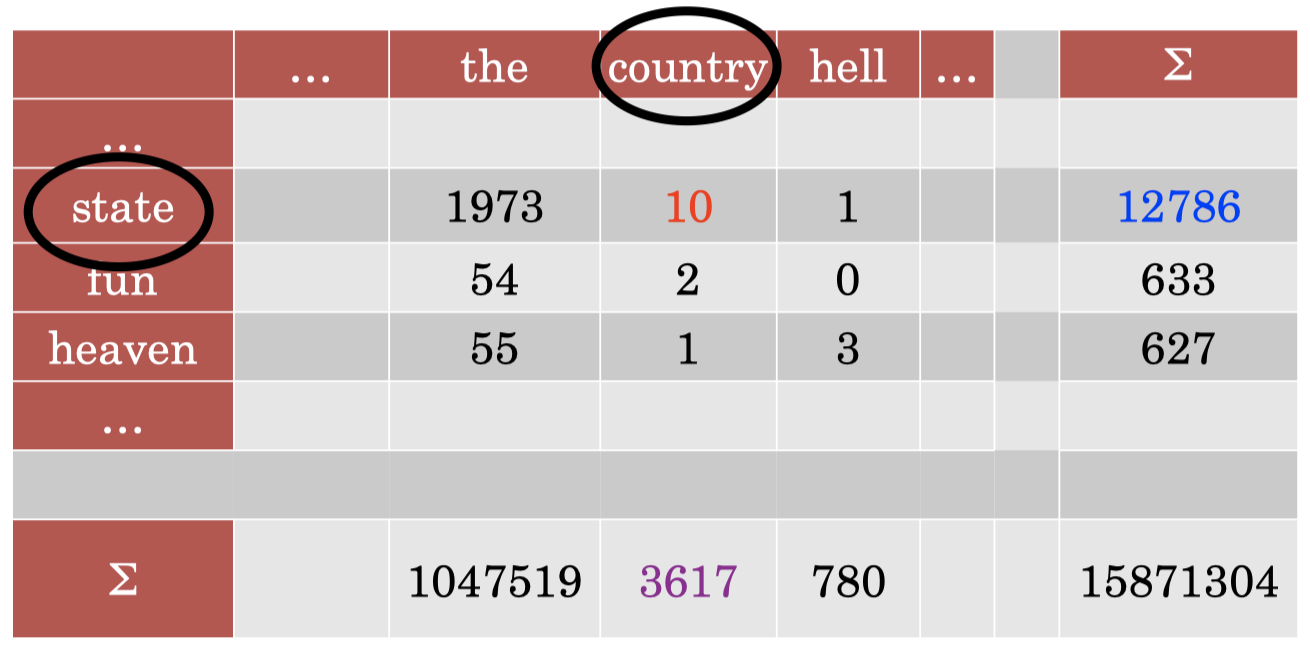\begin{align} p(x,y) &= \text{count}(x,y)/\Sigma \\\\ p(x) &= \Sigma_x/\Sigma \\\\ p(y) &= \Sigma_y/\Sigma \end{align}

\begin{align} p(x,y) &= 10/15871304=6.3 \times 10^{-7} \\\\ p(x) &= 12786/15871304=8.0 \times 10^{-4} \\\\ p(y) &= 3617/15871304=2.3 \times 10^{-4}\\\\ \textit{PMI}(x,y) &= \log_2 \dfrac{6.3 \times 10^{-7}}{8.0 \times 10^{-4}\times 2.3 \times 10^{-4}} = 1.78 \end{align}

### 2.10 PMI 矩阵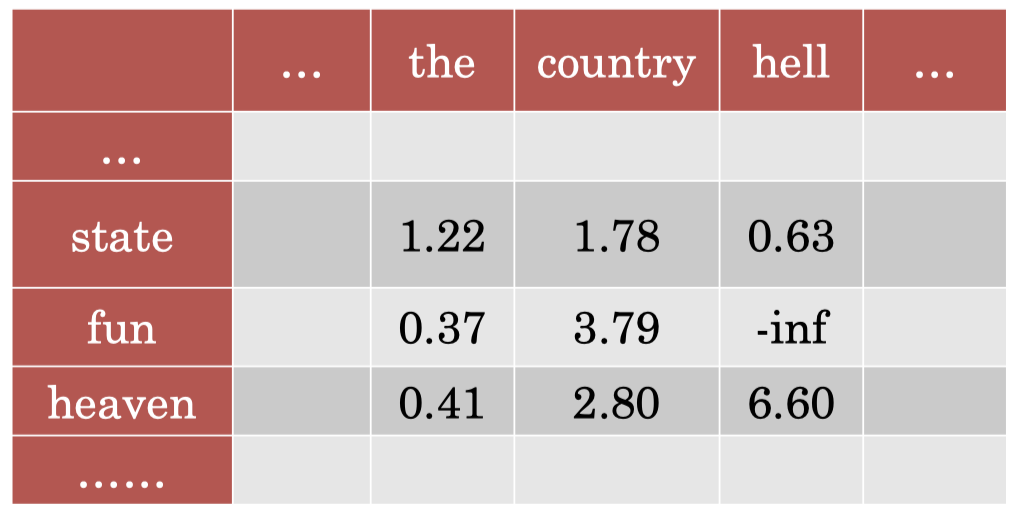• 可以看到，PMI 在捕获我们感兴趣的语义方面做得更好。
• 例如：单词 “$\textit{the}$” 和其他单词之间的 PMI 都很小，单词 “$\textit{heaven}$” 和 “$\textit{hell}$” 之间的 PMI 值较大，因为二者在语义上很接近。
• 但是很明显，这种方法会偏向于给予低频单词较大的 PMI 值。
• 因为根据 PMI 计算公式，其分母部分为 $p(x)p(y)$。对于低频单词，其概率很小，这会导致计算 PMI 时分母变得非常小，从而导致很大的 PMI 值。
• 并且，无法很好地处理频率为 $0$ 的情况（会得到 $-\infty$ 的 PMI 值）。

### 2.11 PMI 技巧

• 将所有负值取 $0$（PPMI）
• 避免了 $-\text{inf}$ 和不可靠的负值，该方法在实践中效果很好。
• 处理罕见事件的偏见问题
• 方法类似之前 n-grams 中的平滑概率。

### 2.12 SVD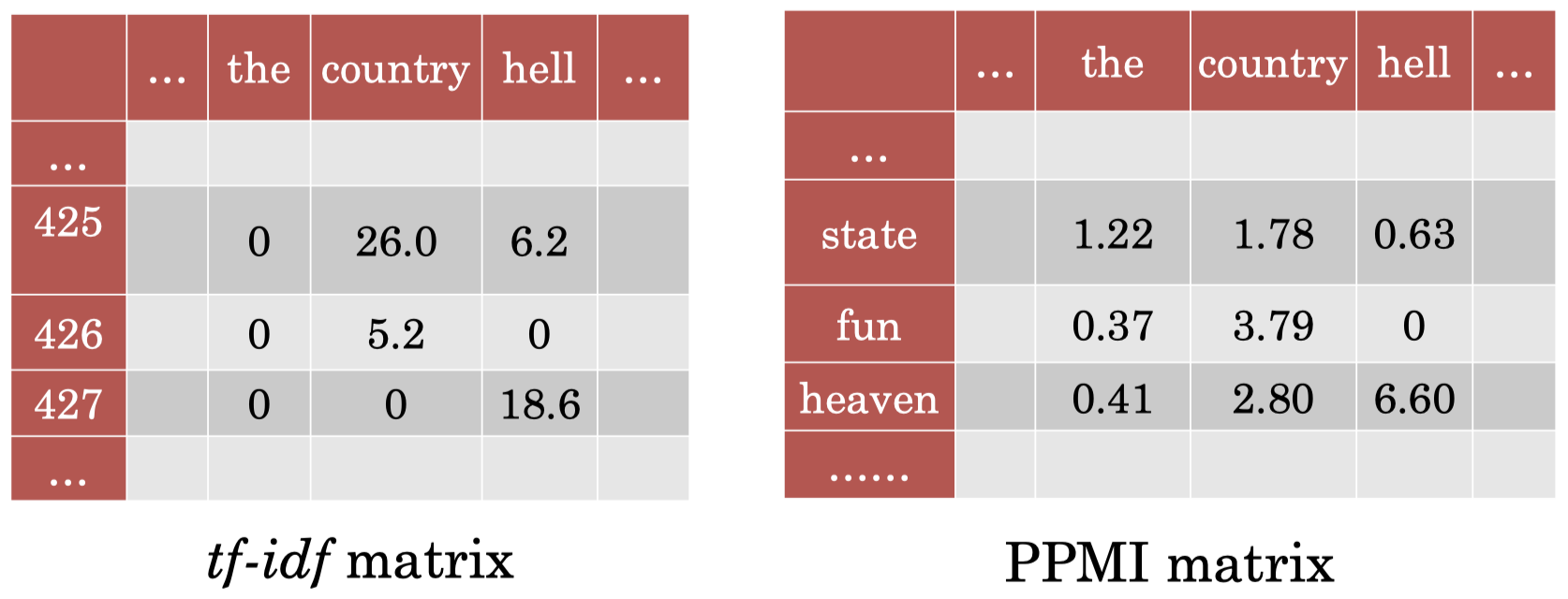### 2.12 相似度

• 词相似度（Word similarity）$=$ 词向量之间的比较（例如：余弦相似度，cosine similarity）。
• 根据向量空间中的 邻近度（proximity）查找 同义词（synonyms）
• 自动构建词汇资源
• 将词向量作为分类器中的特征：相比其他输入形式（例如词频等）更具鲁棒性。
• 例如：$\textit{movie}$ vs. $\textit{film}$ 二者的词向量距离实际上非常近。

## 3. 神经网络方法

### 3.1 词嵌入

• 在之前章节中，我们已经见过神经网络（前馈或循环）使用的 词嵌入 (word embeddings)
• 但是这些模型是为其他任务设计的：
• 分类
• 语言模型
• 词嵌入只是这些模型的一部分。

### 3.2 用于嵌入的神经网络模型

• 我们可以设计专用于学习词嵌入的神经网络吗？
• 理想模型：
• 无监督
我们希望该模型是无监督的，因为有监督模型需要标签信息，而标签信息需要依赖语言学专家进行手工标注。
• 高效
我们希望模型是高效的，因此，我们可以很容易地将其扩展到非常大的语料库上。
• 有用的表示
最重要的是，我们希望学习到的词嵌入能够用于各种下游任务中。

### 3.3 Word2Vec

• 神经网络启发了一些方法，试图学习单词及其上下文的向量表示。
• 关键思想：
• 目标单词的嵌入应与其 相邻单词 的嵌入 相似
• 并且应当与不会出现在其附近的其他单词的嵌入 不相似
• 在训练阶段，将向量点积用于向量 “比较”。
• $\mathbf u\cdot \mathbf v=\Sigma_j \mathbf u_j \mathbf v_j$
• 在测试阶段，我们仍然采用余弦相似度进行比较。
• Word2Vec 的框架是学习一个分类器，有以下 2 种算法：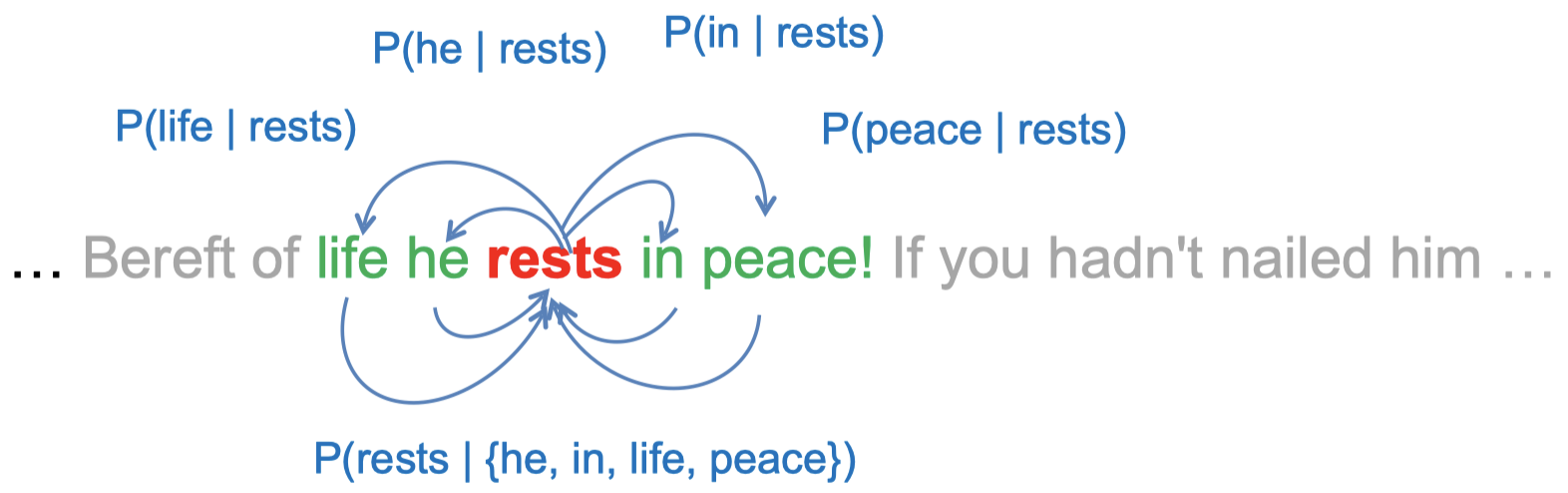• Skip-gram ：给定目标单词，预测该单词周围的局部上下文单词。
• CBOW：给定目标单词周围的局部上下文单词，预测位于中心的目标单词。

两种算法的学习过程基本上是一样的，但是前提条件略有差别，二者之间互为逆过程。

• 局部上下文是指和目标单词距离 $L$ 个位置以内的单词，在上图的例子中，$L=2$。

### 3.4 Skip-gram 模型

• 根据给定的中心单词，生成相应的上下文单词。• 全概率被定义为：

$\prod_{l\in -L,\dots,-1,1,\dots,L}P(w_{t+l}\mid w_t)$

其中，$w_t$ 是目标单词（中心单词），$w_{t+l}$ 是上下文单词，下标表示运行文本中的位置。

• 使用一个 Logistic 回归模型来计算上面每一个乘积项（例如，$w_k=\textit{he},\,w_j=\textit{rests}$）：

$P(w_k\mid w_j)=\dfrac{\exp(\mathbf c_{w_k}\cdot \mathbf v_{w_j})}{\sum_{w'\in V}\exp(\mathbf c_{w'}\cdot \mathbf v_{w_j})}$

其中，$\mathbf c_{w_k}$ 和 $\mathbf v_{w_j}$ 分别表示单词 $w_k$ 和 $w_j$ 的词嵌入。

### 3.5 嵌入参数化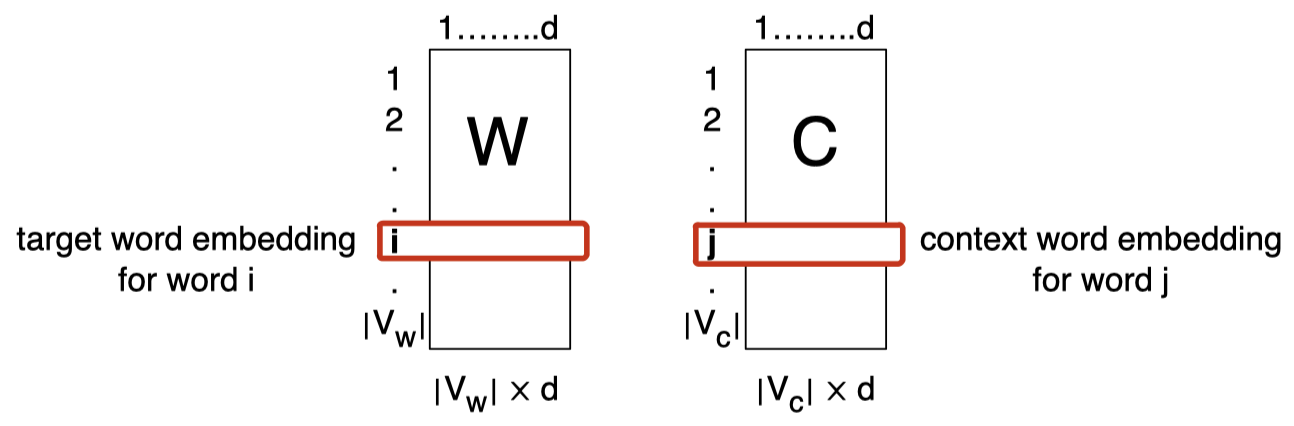### 3.6 Skip-gram 模型的表示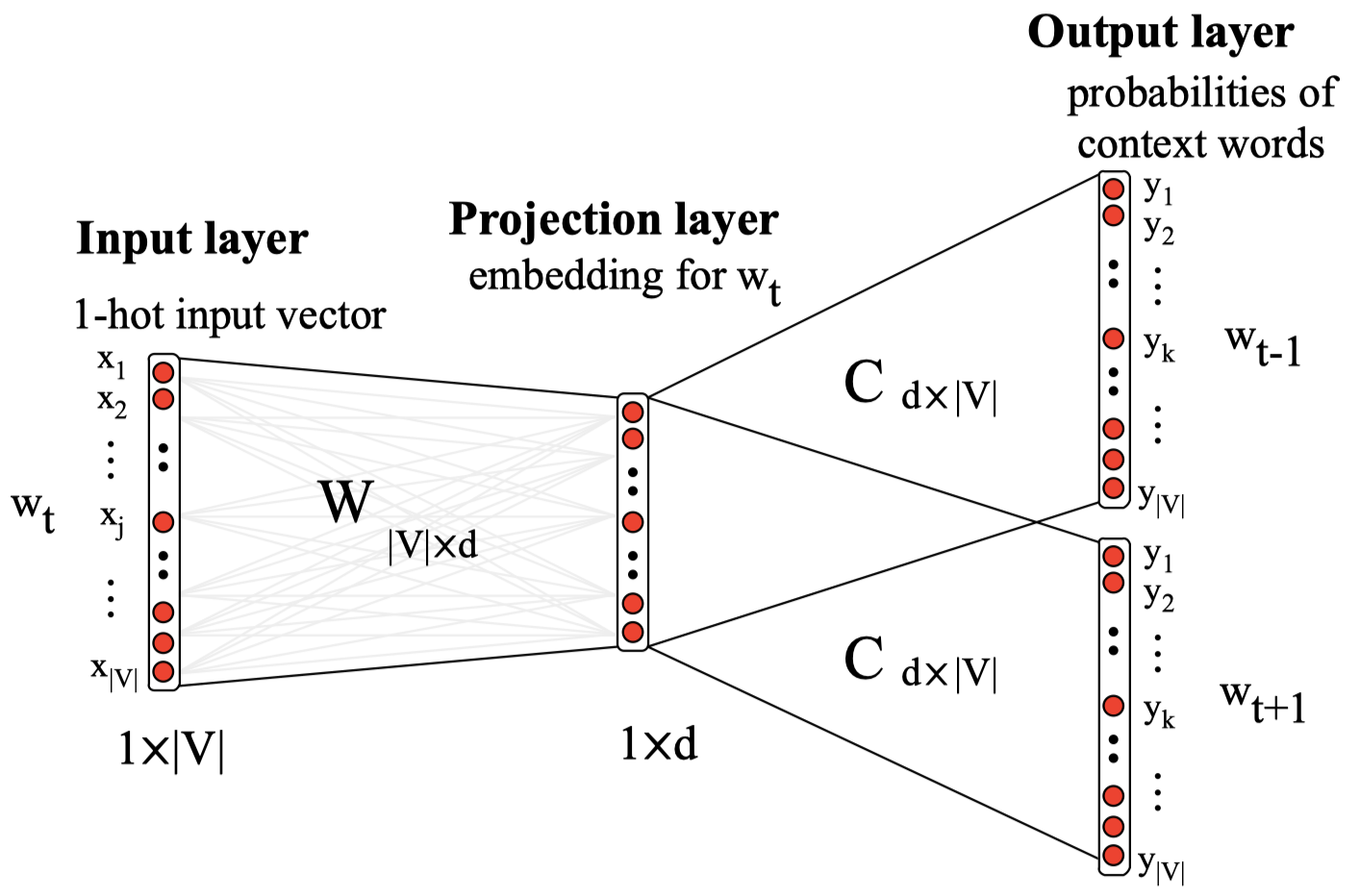### 3.7 训练 Skip-gram 模型

• 训练目标是最大化 原始文本（raw text）的似然。
• 实践中非常缓慢，因为我们需要在整个词汇表 $|V|$ 上进行 归一化（normalisation）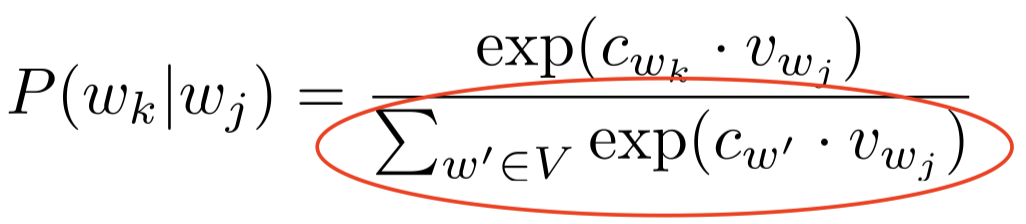当我们在给定目标单词的情况下，计算一个上下文单词的概率时，我们需要将词汇表中所有单词对应的词嵌入的点积进行累加，这个过程非常缓慢，因为在实践中，我们的词汇表可能包含 10,000 甚至 100,000 个单词，这样会使得词汇表的维度变得非常大。

• 因此，Skip-gram 模型的核心是将问题简化为二分类：相比直接进行 $\text{softmax}$ 的相关计算，这里我们只是将真实上下文单词和非上下文单词，又称为 “负样本（negative samples）”，区分开来。
• 这些负样本单词是从词汇表 $V$ 中随机抽取得到的。
因为词汇表通常非常大，所以从词汇表中随机抽样得到真实上下文单词的概率是非常低的。因此，我们可以假设随机抽样得到的单词都是负样本。

### 3.8 负采样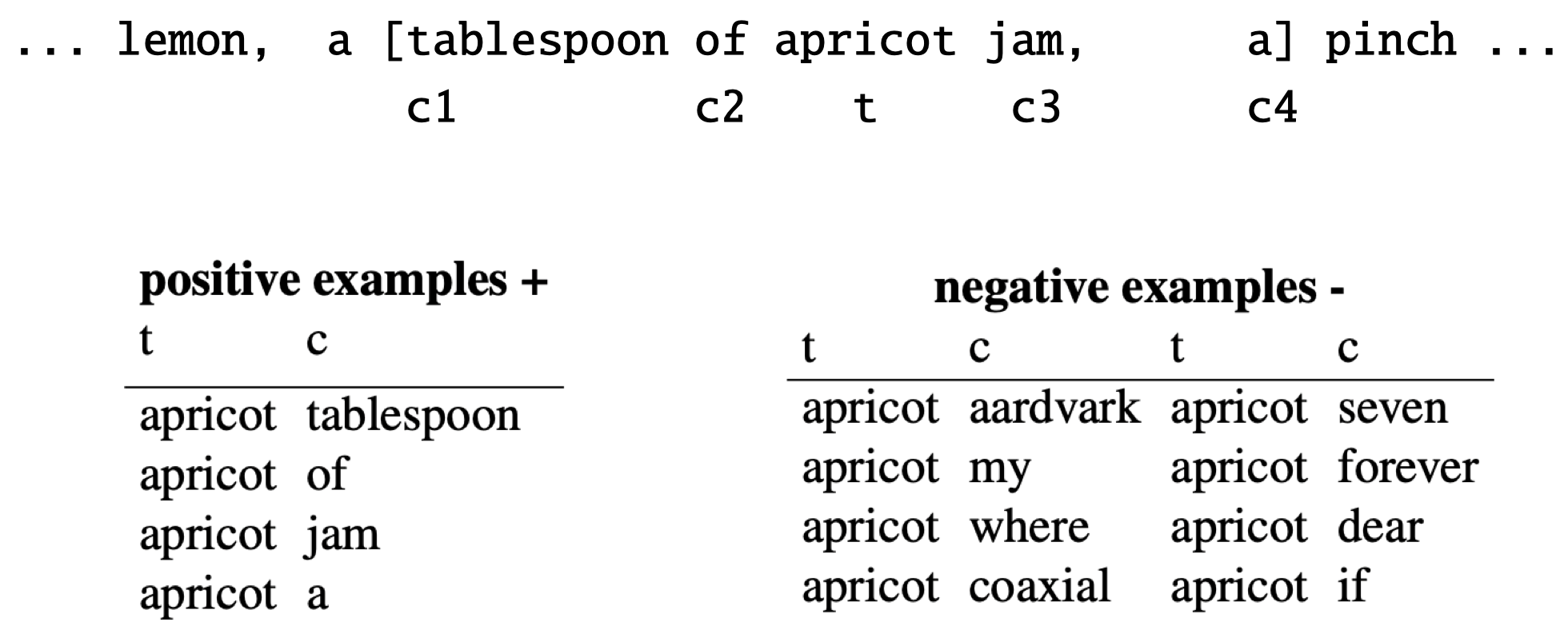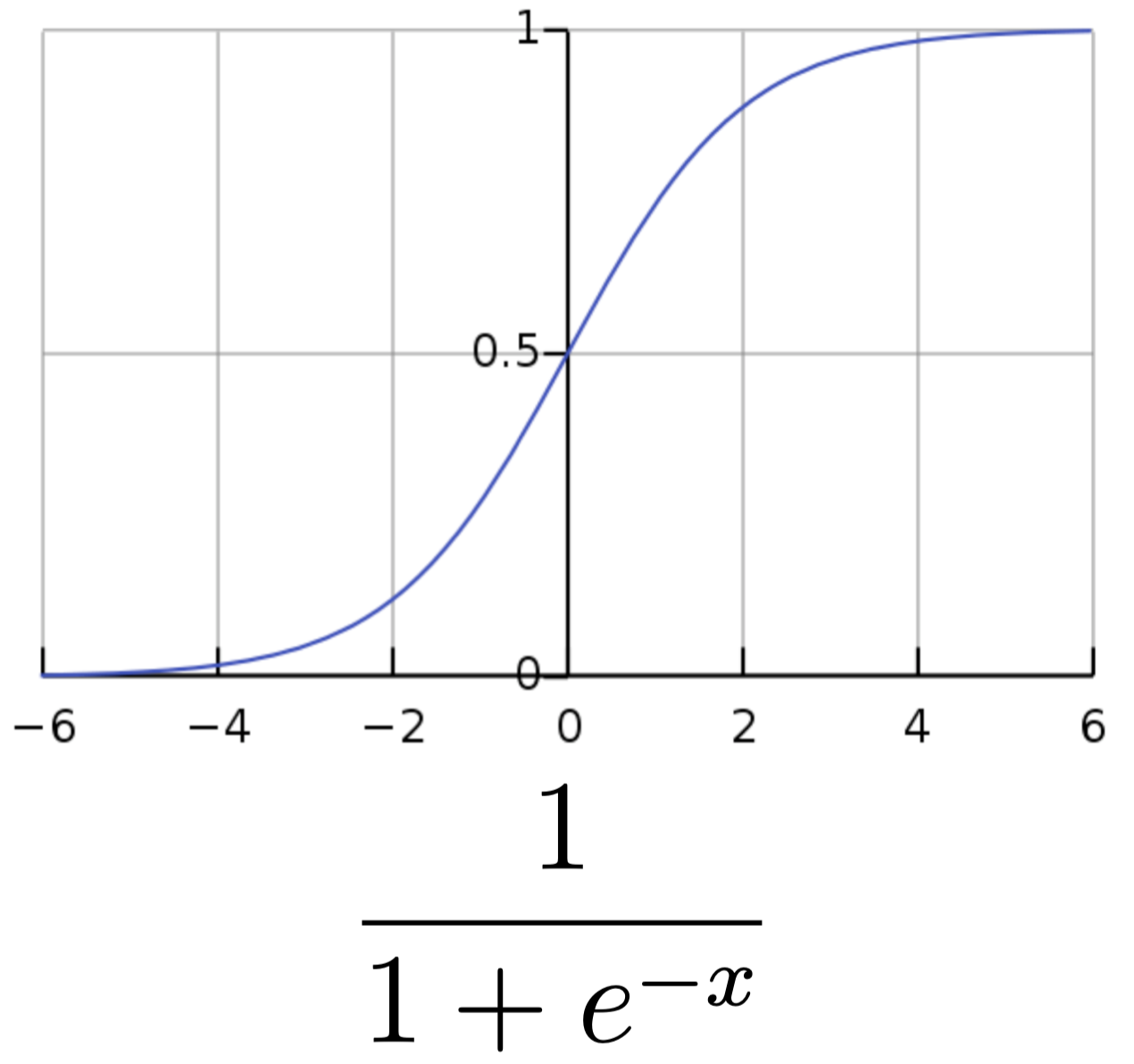\begin{align} P(+\mid t,c) &= \dfrac{1}{1+e^{-t\cdot c}}\\\\ P(-\mid t,c) &= 1-\dfrac{1}{1+e^{-t\cdot c}} \end{align}

### 3.9 Skip-gram 模型的损失函数

$L(\boldsymbol \theta)=\sum_{(t,c)\in +}\log P(+\mid t,c)+\sum_{(t,c)\in -}\log P(-\mid t,c)$

$L(\boldsymbol \theta)=\log P(+\mid t,c)+\sum_{i=1}^k\log P(-\mid t,n_i)$

### 3.10 训练说明

• 在每个迭代步中，我们尝试利用正样本来将目标单词和真实上下文单词的嵌入向彼此靠近的方向移动。这是由正样本的概率提供的。
例如：“$\textit{apricot}$” 和 “$\textit{jam}$”，在每个迭代步中，我们都尝试减小二者的词嵌入之间的距离，让二者更加靠近，即增加二者词嵌入之间的相似度。

• 对于随机采样得到的单词（即负样本），我们希望其和目标单词的嵌入之间尽量拉远距离。
例如：“$\textit{apricot}$” 和 “$\textit{aardvark}$”，我们希望二者词嵌入之间的距离尽可能远。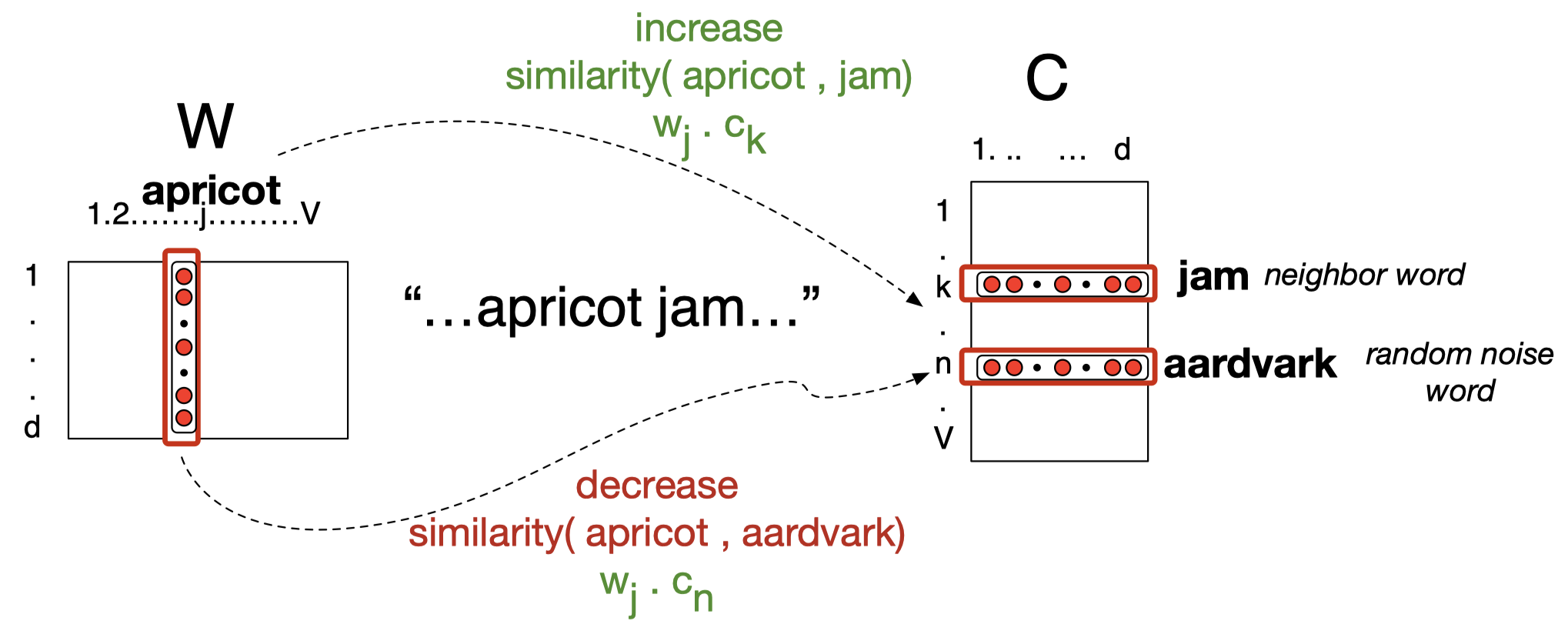### 3.11 理想模型

• 无监督
• 原始的、无标签的语料库
正如前面所述，我们的模型无需标签，只要从给定的语料库按照窗口大小对其中出现的单词进行计数，并计算概率，进行学习即可。
• 高效
• 负采样（避免在整个词汇表上计算 $\text{softmax}$）
• 可以扩展到非常大的语料库上
还有一些在非常大的语料库上预训练的词向量，我们也可以直接下载使用。
• 有用的表示
• 我们如何评估得到的词向量？

### 3.12 评估词向量

• 词典风格任务
我们可以搜集一些单词对，然后让人们对其相似度进行评分，例如：让人们评价单词 $\textit{film}$ 和 $\textit{movie}$ 之间的相似度，或者 $\textit{film}$ 和 $\textit{flower}$ 之间的相似度，并给出一个 0-10 之间的评分。通过收集大量这样的关于单词相似度的评分，我们可以构建出一些标准。

• WordSim-353 是一个由带有相关性评分的名词对组成的数据集。
• SimLex-999 还包含了动词和形容词。
• TOEFL 要求将最接近的同义词作为多选题。
• $\dots$
• 使用向量空间中的余弦相似度测试单词对的兼容性。
我们可以计算得到的词向量之间的余弦相似度，然后将其和人类评分的结果进行对比，例如：计算 Pearson 相关度。如果我们的计算的余弦相似度和人类评分二者的 Pearson 相关系数很高，则说明我们的词嵌入至少和人类从直觉上对单词相似度的判断接近。

### 3.13 嵌入展示有意义的几何关系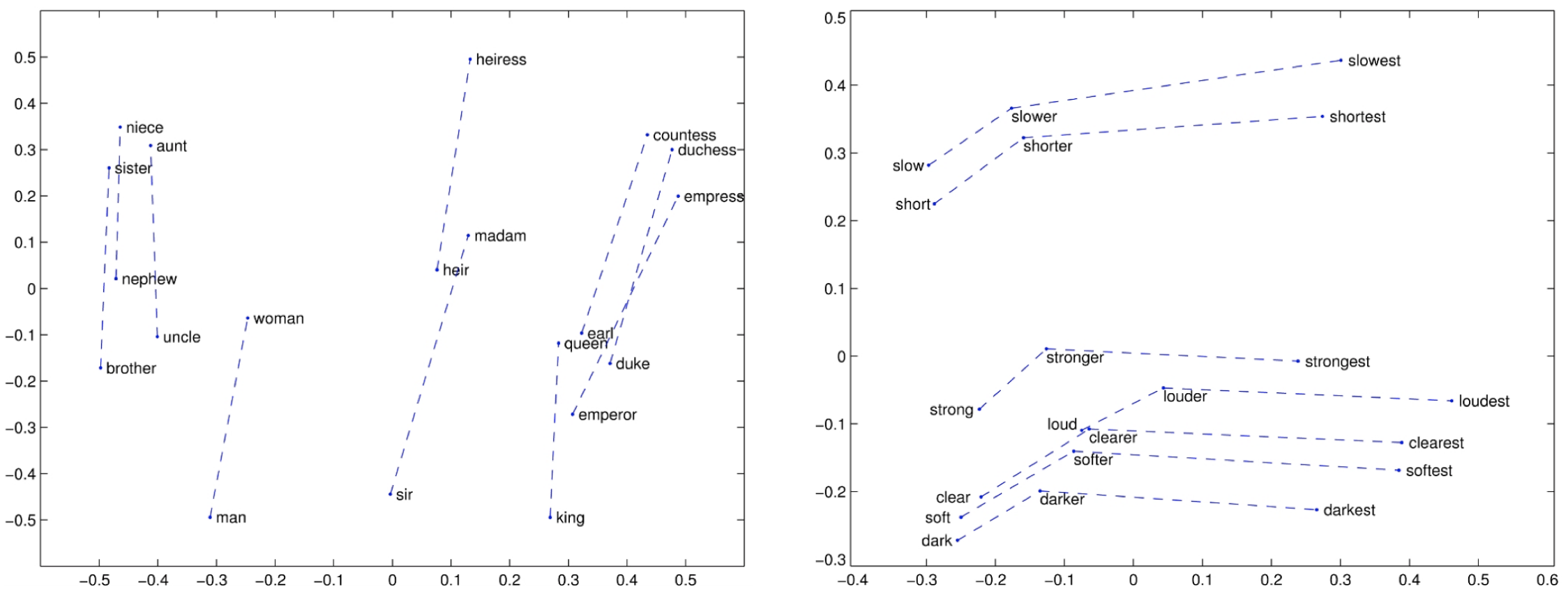• $\textit{Man}$ 对应 $\textit{King}$ 正如 $\textit{Woman}$ 对应 $???$
• $v(\textit{Man})-v(\textit{King})=v(\textit{Woman})-v(???)$
• $v(???)=v(\textit{Woman})-v(\textit{Man})+v(\textit{King})$
• $v(???)=v(\textit{Queen})$
• $???=\textit{Queen}$

### 3.14 评估词向量

• 对于词向量，最佳评估方式是基于其他下游任务中的表现进行评估。
• 使用词袋嵌入作为分类器中的特征表示。
• 大部分深度学习模型中的第一层是对输入文本进行嵌入表示；相比随机初始化所有权重系数，我们可以仅对部分参数进行随机初始化，而对于词嵌入层的初始权重，我们可以使用预训练的词向量作为嵌入的初始化，因为这样我们可以在少量数据上训练出相对准确的词嵌入。
• 最近的 上下文词向量（contextual word vectors）显示在神经网络上具有更好的效果。
• ELMO 和 BERT

例如：根据单词 “$\textit{dog}$” 出现的地方，我们会得到不同的词向量。从句子 1 得到的单词 “$\textit{dog}$” 的词嵌入和从句子 2 得到的单词 “$\textit{dog}$” 的词嵌入可能差别很大，因为二者的上下文不同。BERT 在捕获这种上下文词向量上做得非常好。

## 4. 扩展阅读

• JM3, Ch 6本作品采用知识共享署名-非商业性使用-相同方式共享 4.0 国际许可协议进行许可。 欢迎转载，并请注明来自：YEY 的博客 同时保持文章内容的完整和以上声明信息！